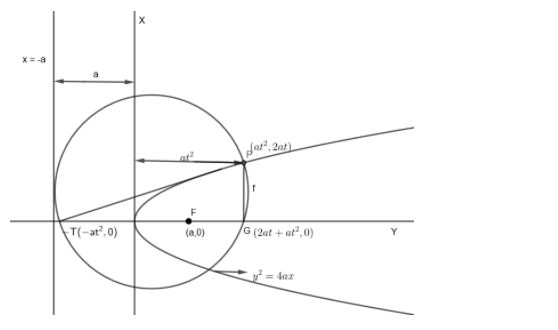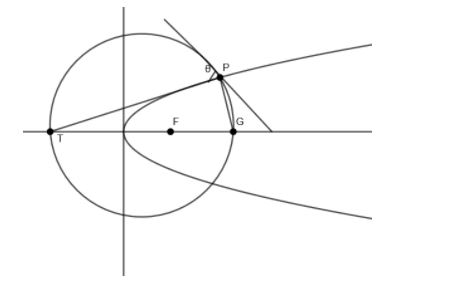QuestionAnswers

# The tangent and normal at P(t), for all real positive t, to the parabola ${{y}^{2}}=4ax$ meet the axis of the parabola in T and G respectively, then the angle at which the tangent at P to the parabola is inclined to the tangent at P to the circle passing through the points P, T and G is:(a) ${{\cot }^{-1}}t$(b) ${{\cot }^{-1}}{{t}^{2}}$(c) ${{\tan }^{-1}}t$(d) ${{\tan }^{-1}}{{t}^{2}}$

Hint: We can start by taking point P $\left( a{{t}^{2}},2at \right)\approx \left( x,y \right)$ on the parabola, then finding slope of tangent as ${{m}_{T}}=\dfrac{y-{{y}_{1}}}{x-{{x}_{1}}}=\dfrac{2at-0}{a{{t}^{2}}-0}=\dfrac{2at}{a{{t}^{2}}}=\dfrac{\Delta y}{\Delta x}$ . Slope of normal would be negative of the reciprocal of slope of tangent as they are perpendicular lines. Now, using equation y=mx+c, we will substitute slope and get the equation of tangent. Using points $\left( a{{t}^{2}},2at \right)\approx \left( x,y \right)$ find, c. Then the values of x, y will get us the coordinates of T. Similarly, we will find coordinates of point G also. Use the distance of the points P, T and G connecting to the focus |PF| = |TF| = |GF| and find the angle connecting the slope of the circle and tangent.

We know that equation of parabola ${{y}^{2}}=4ax$. Let P be the point where the tangent meets the parabola
The tangent meets the x axis at point T and the normal at point G.
Let point P be $\left( a{{t}^{2}},2at \right)\approx \left( x,y \right)$.The slope of the tangent can be found by using,
${{m}_{T}}=\dfrac{y-{{y}_{1}}}{x-{{x}_{1}}}=\dfrac{2at-0}{a{{t}^{2}}-0}=\dfrac{2at}{a{{t}^{2}}}=\dfrac{\Delta y}{\Delta x}$
${{m}_{T}}=\dfrac{2a}{2at}=\dfrac{1}{t}$
The slope of the normal will be $=-\left( \dfrac{1}{{{m}_{T}}} \right)=-\left( \dfrac{1}{\dfrac{1}{t}} \right)=-t$
$\therefore {{M}_{n}}=-t$
We know the equation of tangent,
$\Rightarrow y=mx+c....\left( i \right)$
We got the slope m as $\left( \dfrac{1}{t} \right)$ for the tangent.
Therefore, equation (ii) of the tangent changes to
$\Rightarrow y=\dfrac{x}{t}+c....\left( ii \right)$
We know the value of $y=2at$ and $x=a{{t}^{2}}$.
Substituting these values in equation (ii) along with slope $m=\dfrac{1}{t}$.
We get,
$2at=\dfrac{a{{t}^{2}}}{t}+c$
By simplifying it, we get
$2at=at+c$
$\Rightarrow c=2at-at$
$\therefore c=at$
Now, put the value of c in equation (ii).
$\Rightarrow y=\dfrac{x}{t}+at....\left( iii \right)$
Now, put the value of y = 0, to get the coordinates of point T.
$0=\dfrac{x}{t}+at$
$\Rightarrow x=\left( -at \right)t=-a{{t}^{2}}$
Therefore, Coordinates of T are $\left( -a{{t}^{2}},0 \right)$.
We got the slope of Normal, ${{m}_{N}}=-t$.
By putting the value of slope in $y=mx+c$, we get
$y=-tx+c....\left( iv \right)$
Put $\left( x,y \right)=\left( a{{t}^{2}},2at \right)$ in the above equation.
$2at=-t\left( a{{t}^{2}} \right)+c$
$\Rightarrow 2at+a{{t}^{3}}=c$
$\therefore c=at\left( 2+a{{t}^{2}} \right)$
Now, put the value of c in equation (iv)
$y=-tx+at\left( 2+a{{t}^{2}} \right)$
$\Rightarrow y=-tx+2at+a{{t}^{3}}$
Put the value of y = 0, to get the coordinates of point G.
$0=-tx+2at+a{{t}^{3}}$
$\Rightarrow tx=2at+a{{t}^{3}}$
Dividing throughout with ‘t’ on both RHS and LHS
$\dfrac{tx}{x}=\dfrac{2at+a{{t}^{3}}}{t}$
$\Rightarrow x=2a+a{{t}^{2}}$
Therefore, we get the coordinates of G as $\left( 2a+a{{t}^{2}},0 \right)$.
Now, let us take the focus of parabola as F with coordinates (a, 0).
Now, calculate the distance of FT by using the formula
$\sqrt{{{\left( {{x}_{2}}-{{x}_{1}} \right)}^{2}}+{{\left( \dfrac{1}{2}-\dfrac{1}{1} \right)}^{2}}}$
Therefore, distance of |FT| where $F\left( a,0 \right)$ and $T\left( -a{{t}^{2}},0 \right)$.
$\left| FT \right|=\sqrt{{{\left[ a-\left( -a{{t}^{2}} \right) \right]}^{2}}+\left[ 0-0 \right]}=\sqrt{{{\left( a+a{{t}^{2}} \right)}^{2}}}=a+a{{t}^{2}}$
Similarly distance between |FG| where $F\left( a,0 \right)$ and $G\left( 2a+a{{t}^{2}},0 \right)$.
$\left| FG \right|=\sqrt{\left( 2a+a{{t}^{2}}-{{a}^{2}} \right)+\left( 0-0 \right)}=\sqrt{{{\left( a+a{{t}^{2}} \right)}^{2}}}=a+a{{t}^{2}}$
Similarly, if we find the distance of |PF|
Therefore, the directrix of parabola will be x + a = 0 from x axis and then $\left( x-a{{t}^{2}} \right)$ to point p on the other side of x – axis.
Therefore from the figure, the distance $\left| PF \right|=a+a{{t}^{2}}\text{ }$
Now, we can create the circle connecting the points T, P and G.
So focus F becomes the center of the circle.
We know that the equation of the circle,
${{\left( x-g \right)}^{2}}+{{\left( y-f \right)}^{2}}={{r}^{2}}....\left( v \right)$
where (g, f) is the center of the circle.
Substituting, (g, f) as (a, 0) in equation (v), we get
${{\left( x-a \right)}^{2}}+{{\left( y-0 \right)}^{2}}={{\left( a+a{{t}^{2}} \right)}^{2}}$
where radius, $r=a+a{{t}^{2}}$
Now, differentiate ${{\left( x-a \right)}^{2}}+{{y}^{2}}={{\left( a+a{{t}^{2}} \right)}^{2}}$, we get
$2\left( x-a \right)+2y.\dfrac{dy}{dx}=0$
By deriving the value of $\dfrac{dy}{dx}=\dfrac{-2\left( x-a \right)}{2y}$
$\therefore \dfrac{dy}{dx}=\dfrac{-\left( x-a \right)}{y}=\dfrac{a-x}{y}$
Substitute the value of x and y in the above equation,
i.e. $\left( x,y \right)=\left( a{{t}^{2}},2at \right)$
$\therefore \dfrac{dy}{dx}=\dfrac{a-a{{t}^{2}}}{2at}=\dfrac{a\left( 1-{{t}^{2}} \right)}{2at}=\dfrac{1-{{t}^{2}}}{2t}={{m}_{2}}$
i.e the slope of the circle.
Now, let us draw a tangent on the circle whose slope is equal to the tangent through P. The angle between the new tangent and circle will be $\theta$. Here focus is taken as the center of the circle formed by points P, G and T. The focus is at the intersection of the axis and the circle. Therefore, it becomes the center of the circle.$\therefore \tan \theta =\left| \dfrac{{{m}_{1}}-{{m}_{2}}}{1+{{m}_{1}}{{m}_{2}}} \right|$
This is to find the angle made by the tangent and the circle.
We know the slope, ${{m}_{1}}=\dfrac{1}{t}$, i.e. the slope of the tangent.
The slope of the circle, ${{m}_{2}}=\dfrac{1-{{t}^{2}}}{2t}$
$\therefore \tan \theta =\left| \dfrac{\dfrac{1}{t}-\left( \dfrac{1-{{t}^{2}}}{2t} \right)}{1+\left( \dfrac{1}{t} \right)\left( \dfrac{1-{{t}^{2}}}{2t} \right)} \right|=\left| \dfrac{\dfrac{2-1+{{t}^{2}}}{2t}}{1+\dfrac{1-{{t}^{2}}}{2{{t}^{2}}}} \right|$
$=\left| \dfrac{1+\dfrac{{{t}^{2}}}{2t}}{\dfrac{2{{t}^{2}}+1-{{t}^{2}}}{2{{t}^{2}}}} \right|=\left| \dfrac{1+{{t}^{2}}}{1+\dfrac{{{t}^{2}}}{t}} \right|=\left| \dfrac{t\left( 1+{{t}^{2}} \right)}{1+{{t}^{2}}} \right|$
$\therefore \tan \theta =t$
$\therefore \theta ={{\tan }^{-1}}t$

So, the correct answer is “Option C”.

Note: The directrix of parabola is taken as x + a = 0 and the center of the circle is taken as the focus with (a, 0). For this question, we must understand that we are supposed to find the slope of a circle and the slope of a tangent to find the angle. We must draw the figure properly to understand that distance PF is the radius of circle and then differentiate the equation of circle obtained after substituting in general form of circle to get slope of circle.